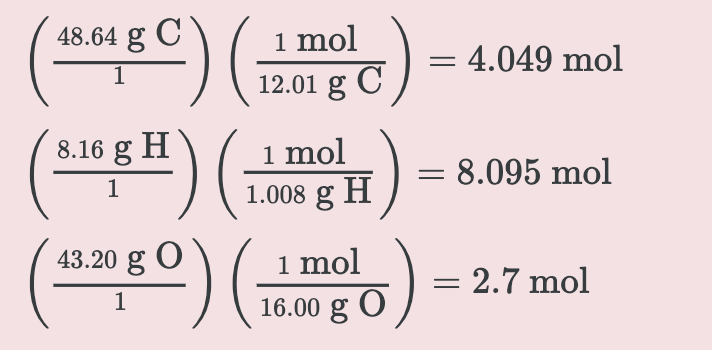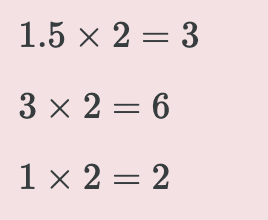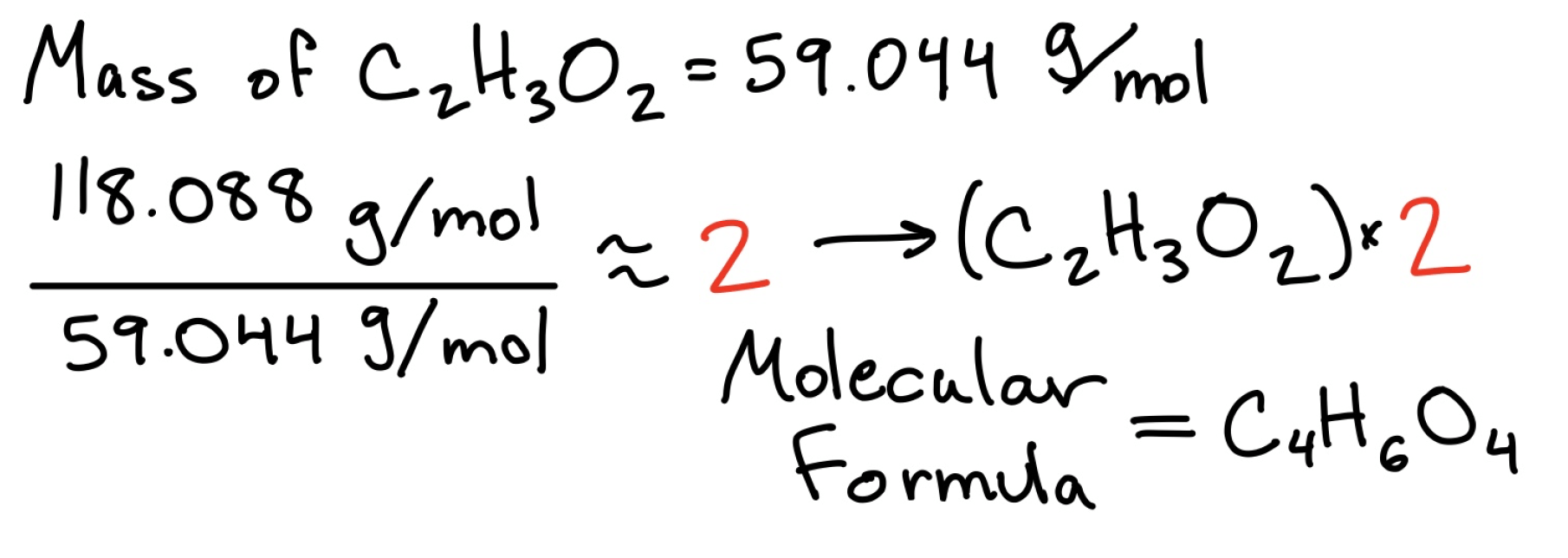Cyber Monday Sale: ALL MCAT Products & Admissions Services

MCAT Content / Stoichiometry / Empirical Versus Molecular Formula

### Empirical versus Molecular Formula

Topic: Stoichiometry

The molecular formula indicates the actual number of atoms in a compound; The empirical formula indicates the smallest whole-number ratio of atoms in a compound.

The molecular formula for a compound such as phosphorous pentoxide is P4O10. We can see this as a ratio of 4 phosphorus atoms to 10 oxygen atoms, which can be written as 4:10. This ratio can be reduced to 2:5, as both numbers are divisible by 2. Therefore, the empirical formula of P4O10 can be written as P2O5.

Empirical formulas can be determined using mass composition data. If we know the mass or percentage composition of each element in a compound, this information can be converted into moles and then used to work out the empirical formula.

Suppose you are given a compound such as methyl acetate, a solvent commonly used in paints, inks, and adhesives. When methyl acetate was chemically analyzed, it was discovered to have 48.64% carbon (C), 8.16% hydrogen (H), and 43.20% oxygen (O). For the purposes of determining empirical formulas, we assume that we have 100 g of the compound. If this is the case, the percentages will be equal to the mass of each element in grams.

Step 1: Change each percentage to an expression of the mass of each element in grams. That is, 48.64% C becomes 48.64 g C, 8.16% H becomes 8.16 g H, and 43.20% O becomes 43.20 g O because we assume we have 100 g of the overall compound.

Step 2: Convert the amount of each element in grams to its amount in moles by dividing by the molecular weightStep 3: Divide each of the mole values by the smallest of the mole values.Step 4: If necessary, multiply these numbers by integers in order to get whole numbers; if an operation is done to one of the numbers, it must be done to all of them.Thus, the empirical formula of methyl acetate is C3H6O2.

Given the molecular weight of the unknown, the molecular formula of the unknown compound can be determined. If for example our substance has a mass of 118 g/mol and we worked out the molecular formula to be C2H3O2 this would have a mass of 59 g/mol which goes into 118 twice, so I need to multiply through my empirical formula by a factor of 2 to get my molecular formula C4H6O4.Practice Questions

MCAT Official Prep (AAMC)

Chemistry Online Flashcards Question 15

Chemistry Question Pack Question 69

Chemistry Question Pack Passage 15 Question 82

Key Points

• Empirical formulas are the simplest form of notation.

• The molecular formula for a compound is equal to, or a whole-number multiple of, its empirical formula.

• Like molecular formulas, empirical formulas are not unique and can describe a number of different chemical structures or isomers.

• To determine an empirical formula, the mass composition of its elements can be used to mathematically determine their ratio.

Key Terms

Per cent composition: elemental composition by mass per cent

Molecular weight: the total mass of atoms in a molecule, represented by g/mol

Empirical formula: a notation indicating the ratios of the various elements present in a compound, without regard to the actual numbers

Molecular formula: a formula that describes the exact number and type of atoms in a single molecule of a compound

Billing Information